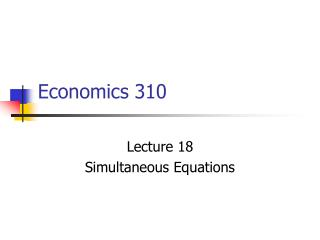DownloadDownload PresentationEconomics 310

# Economics 310

Télécharger la présentation## Economics 310

- - - - - - - - - - - - - - - - - - - - - - - - - - - E N D - - - - - - - - - - - - - - - - - - - - - - - - - - -
##### Presentation Transcript

1. Economics 310 Lecture 18 Simultaneous Equations

2. Simultaneous Equations • There is a two-way, or simultaneous, relationship between Y and (some of) the X’s, which makes the distinction between dependent and explanatory variables of dubious value. • In simultaneous equations there is more than one equation – one for each of the mutually, or jointly, dependent or endogenous variables.

3. Simultaneous Equations • In the simultaneous-equation models one may not estimate the parameters of a single equation without taking into account information provided by other equations in the system. • Jointly dependent variables are called endogenous variables • The explanatory variables that are independent of the error are called exogenous variable or predetermined variables.

4. Example Simultaneous Equations: Demand-Supply

5. P P’ P* Q Q* Q’ Demand Shifts S D’ D*

6. Violation Classic Assumptions • It is clear from drawing that observed values of P and Q depend on the error in the demand equation. • The P in the demand equation is not independent of the equation’s error. • We have a violation of the classic assumptions.

7. Keynesian Model of Income

8. Keynesian Model C,I Y=C+I C+I 45° Y Y0

9. Shift in Consumption C,I Y=C+I C’+I C+I 45° Y Y0 Y1

10. Consumption Function • Income is correlated with error in the consumption function. • We cannot estimate the consumption function by OLS due to violation of classic assumption • Our OLS estimate will suffer from Simultaneous equation bias.

11. Simultaneous equation bias

12. Simultaneous equation bias

13. Simultaneous equation bias

14. Probability Limit and Consistency

15. Simultaneous equation biasProbability Limit

16. Reduced Form equation

17. Reduced Form Equations

18. Indirect least-squares

19. Indirect Least-squares

20. Consistency of Estimates

21. Review Indirect Least Squares • We first established a relationship between the structural and reduced form equations and parameters. • We then estimated the reduced form parameters using the least squares estimation rule. • We then derived estimates of the structural parameters from the estimated reduced form parameters. • We noted that while the direct least squares estimator used to estimated the structural parameters was biased and inconsistent, the indirect least squares estimator, using the estimated reduced form parameters, had the statistical property of consistency.